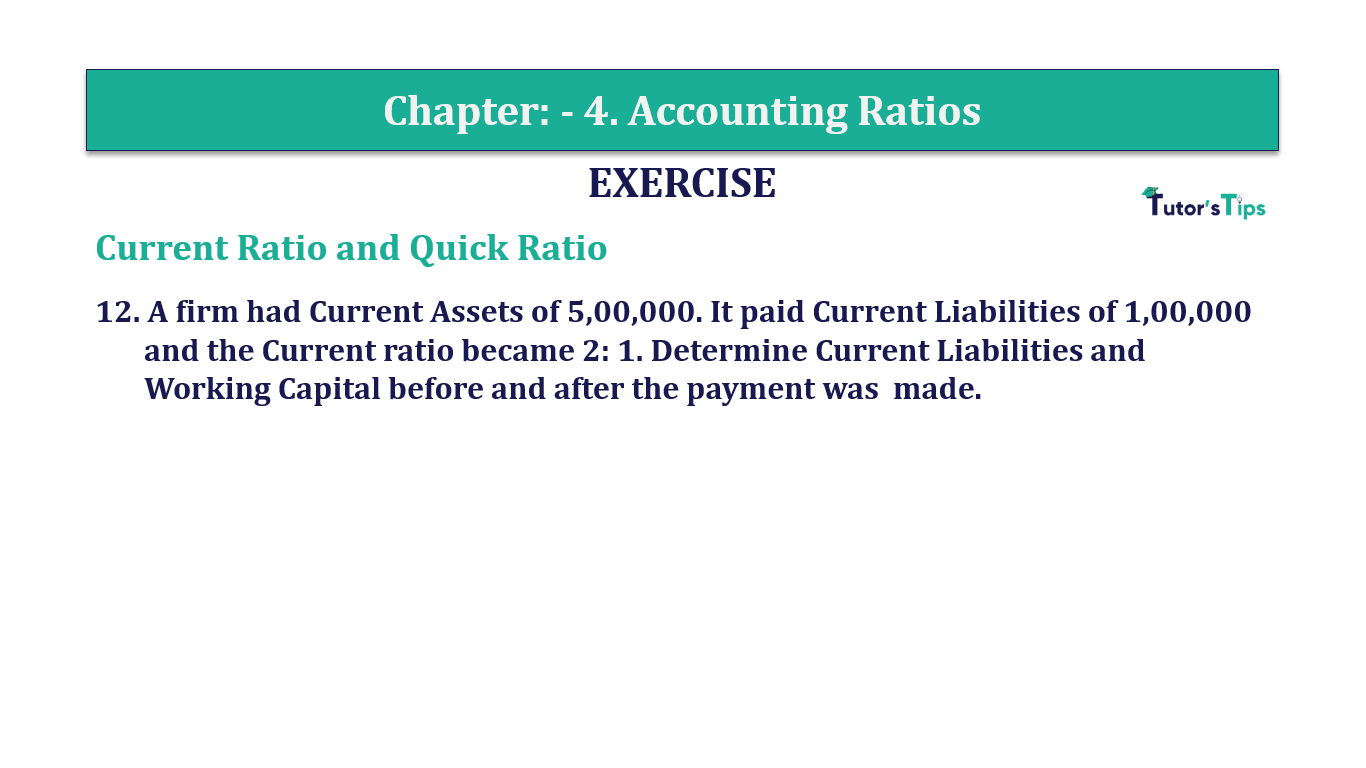# Question 12 Chapter 4 of +2-B – T.S. Grewal 12 ClassQuestion No. 12 - Chapter No.4 - T.S. Grewal +2 Book Part B

Question 12 Chapter 4 of +2-B

Current Ratio and Quick Ratio

12. A firm had Current Assets of 5,00,000. It paid Current Liabilities of 1,00,000 and the Current ratio became 2: 1. Determine Current Liabilities and Working Capital before and after the payment was made.

### The solution of Question 12 Chapter 4 of +2-B: –

The Firm paid off liabilities of Rs.1,00,000
which results in a decrease in current liabilities and current assets by the same amount.
After making the payment:

 Current Assets =Rs.4,00,000 (Rs.5,00,000 – Rs.1,00,000)

∴ Let Current Liabilities be = (x – Rs.1,00,000)

 Current Ratio = Current Assets = Rs. 8,75,000 = 2 Current Liabilities Rs. 3,50,000 1
 Rs. 4,00,000 =2x – Rs. 2,00,000
 Rs. 6,00,000 =2x
 Therefore, x =3,00,000

 Current Liabilities after payment = – Rs.1,00,000 = Rs.2,00,000 Working Capital after Payment = Current Assets – Current Liabilities = Rs.4,00,000 – Rs.2,00,000 =Rs. 2,00,000 Current Assets before payment = Rs.5,00,000 Current Liabilities before Payment = Rs.3,00,000 Therefore, Working Capital Before Payment – Current Assets – Current Liabilities = Rs.5,00,000 – Rs.3,00,000 = Rs.2,00,000

Therefore, goods worth Rs.1,75,000 must be purchased on credit to maintain the current ratio at 2:1

Balance Sheet: Meaning, Format & Examples

Comment if you have any question.

Also, Check out the solved question of previous Chapters: –